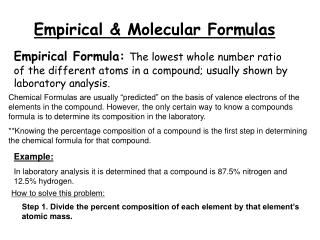DownloadDownload PresentationEmpirical & Molecular Formulas

# Empirical & Molecular Formulas

Télécharger la présentation## Empirical & Molecular Formulas

- - - - - - - - - - - - - - - - - - - - - - - - - - - E N D - - - - - - - - - - - - - - - - - - - - - - - - - - -
##### Presentation Transcript

1. Empirical & Molecular Formulas Chemical Formulas are usually “predicted” on the basis of valence electrons of the elements in the compound. However, the only certain way to know a compounds formula is to determine its composition in the laboratory. **Knowing the percentage composition of a compound is the first step in determining the chemical formula for that compound. Empirical Formula:The lowest whole number ratio of the different atoms in a compound; usually shown by laboratory analysis. Example: In laboratory analysis it is determined that a compound is 87.5% nitrogen and 12.5% hydrogen. How to solve this problem: Step 1. Divide the percent composition of each element by that element’s atomic mass.

2. For Hydrogen:12.5 = 12.4 1.01 Step 2: Express this number as the smallest whole number ratio. To do this, divide both numbers by the smallest one. For Nitrogen: 87.5 = 6.25 14.01 6.25= 1 6.25 12.4 = 1.98 = 2 (nearest whole number) 6.25 The whole number ratio is 1:2 This ratio expresses the ratio of atoms in the compound. The empirical formula for this compound, then, is NH2. Let’s try another: At a crime scene a dark substance is found on the skin of a victim. Forensic experts take a sample of the substance and determine through analysis that is 63.5 % silver, 8.2% nitrogen, and 28.2% oxygen. What is the empirical formula of this compound? Step 1:Divide the percent composition of each element by that element’s atomic mass. Ag:63.5 = 0.589 107.9 N:8.2 = 0.585 14.01 O:28.2 = 1.76 16.00

3. Step 2: Express this number as the smallest whole number ratio. To do this, divide both numbers by the smallest one. 0.589 = 1.006 0.585 0.585 = 1 0.585 1.76 = 3.01 = 3 0.585 (nearest whole number) The whole number ratio is 1:1:3 The whole number ratio is given in the order that the elements are listed. The empirical formula is AgNO3. Empirical Formulas may or may not be the “actual” or real formula of your compound. **If it is an ionic compound, the empirical formula is usually the true formula **If it is a molecular compound, you need to go on to find the Molecular Formula.

4. Molecular Formulas:The molecular formula shows the true number of atoms of each kind of element. It is always a whole number multiple of the empirical formula. In order to determine the molecular formula, you need to know the molar mass (usually determined through experimentation methods). For Example, hydrazine is a compound that contains nitrogen and hydrogen. Its empirical formula is NH2, but its molar mass is 32.0 g/mol. What is the true formula of hydrazine? How to solve this problem: Step 1: Determine the formula mass of the empirical formula 1 N + 2 H = 14.01 + (2 x1.01) = 16.02g/ mol Step 2: Divide the Molar mass by the empirical formula mass (efm). Round to the nearest whole number. This will give you the ‘multiple’ of the empirical formula 32.0 g/mol ÷ 16.02 g/mol = 1.99 = 2 (whole number)

5. Step 3: Multiply the subscripts in the empirical formula by your number calculated in step 2. The molecular formula for hydrazine is N2H4 [ NH2 ] x 2 = N2H4 Let’s try another: Benzene is a molecular compound with a molar mass 78.1 g/mol. The empirical formula is CH. What is the molecular formula of benzene? Step 1: Determine the formula mass of the empirical formula 1 C & 1 H = 12.01 + 1.01 = 13.02 g/mol Step 2: Divide the Molar mass by the empirical formula mass (efm). 78.1 g/mol ÷ 13.02 g/mol = 5.99 = 6 (nearest whole number) Step 3: Multiply the subscripts in the empirical formula by your number calculated in step 2. The molecular formula for benzene is C6H6 [ CH ] x 6 = C6H6